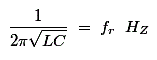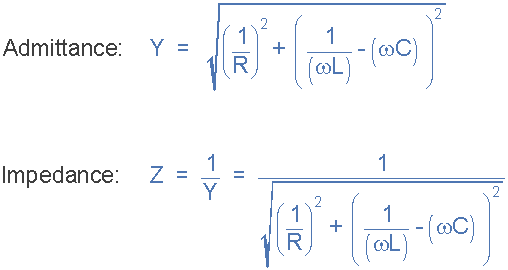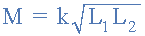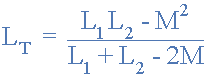# Parallel Mutual Inductances

## Homework Statement

FIGURE 4(a) shows two inductances connected in parallel across an a.c. supply.
(a) Apply Kirchhoff’s voltage law to loop abef and to loop abcdef of the circuit.

(b) Hence or otherwise obtain the current ratio I1/I2 in terms of the circuit inductances.

(c) See if you can show that L1 and L2 can be replaced by the
equivalent inductor, Leq, of FIGURE 4(b) where Leq = (L1*L2-M^2)/(L1+L2-2*M)

(d) A 1 nF capacitor is placed across the two inductors (FIGURE 4 (c)). If L1 = L2 = L and k = 0.5, determine the required value of L if the minimum current I flows from the supply when it is at a frequency of 1 MHz.

## The Attempt at a Solution

(a) ABEF : V=jw*L1*I1+jw*M*I2, ABCDEF : V=jw*L2*I2+jw*M*I1

(b) I1= (V*(L2-M)) / (jw*(L1*L2-M^2)) , I2=(V*(L1-M)) / (jw*(L1*L2-M^2))

I1/I2= (((V*(L2-M)) / (jw*(L1*L2-M^2))) / (((V*(L1-M)) / (jw*(L1*L2-M^2)))

I1/I2 =L2-M / L1-M

(c) Leq = I1+I2 , = (((V*(L2-M)) / (jw*(L1*L2-M^2))) + (((V*(L1-M)) / (jw*(L1*L2-M^2)))

=(V*(L1+L2-2M))/(jw*(L1*L2-M^2)) = V/jw*Leq, Leq = (L1*L2-M^2)/(L1+L2-2*M)

(d) Xc= 1/(2*pi*f*C) = j159.155 ohms
The bit i'm stuck on is whether I can solve for L using :However this is for resonant frequency. A push in the right direction anyone on any of these questions. Can someone explain the origin of why in (c) I1+I2= V/jw*Leq

gneill
Mentor

## Homework Statement

FIGURE 4(a) shows two inductances connected in parallel across an a.c. supply.
(a) Apply Kirchhoff’s voltage law to loop abef and to loop abcdef of the circuit.

(b) Hence or otherwise obtain the current ratio I1/I2 in terms of the circuit inductances.

(c) See if you can show that L1 and L2 can be replaced by the
equivalent inductor, Leq, of FIGURE 4(b) where Leq = (L1*L2-M^2)/(L1+L2-2*M)

(d) A 1 nF capacitor is placed across the two inductors (FIGURE 4 (c)). If L1 = L2 = L and k = 0.5, determine the required value of L if the minimum current I flows from the supply when it is at a frequency of 1 MHz.

## The Attempt at a Solution

(a) ABEF : V=jw*L1*I1+jw*M*I2, ABCDEF : V=jw*L2*I2+jw*M*I1

(b) I1= (V*(L2-M)) / (jw*(L1*L2-M^2)) , I2=(V*(L1-M)) / (jw*(L1*L2-M^2))

I1/I2= (((V*(L2-M)) / (jw*(L1*L2-M^2))) / (((V*(L1-M)) / (jw*(L1*L2-M^2)))

I1/I2 =L2-M / L1-M

(c) Leq = I1+I2 , = (((V*(L2-M)) / (jw*(L1*L2-M^2))) + (((V*(L1-M)) / (jw*(L1*L2-M^2)))
That should be ##I = I_1 + I_2##, the sum of the currents. Current and inductance are completely different animals.
=(V*(L1+L2-2M))/(jw*(L1*L2-M^2)) = V/jw*Leq, Leq = (L1*L2-M^2)/(L1+L2-2*M)

(d) Xc= 1/(2*pi*f*C) = j159.155 ohms
The bit i'm stuck on is whether I can solve for L using :However this is for resonant frequency.
The inductor and capacitor are in parallel, so it would make sense to view this load as parallel admittances. No current will flow if the total admittance is zero, right? How do admittances in parallel combine? You should be able to easily show that the total admittance is zero at resonance.
A push in the right direction anyone on any of these questions. Can someone explain the origin of why in (c) I1+I2= V/jw*Leq
In general the steady state current through an inductance L due to applied potential V is ##I = V/jωL##. In this problem I1 + I2 is the current total ##I## for the load, and V is the potential across the load. So knowing V and I you can determine an equivalent inductance for the load provided that the current has the same form.Yt=Y+Y+Y...

wC=j0.006 , Yt=0 , 0= 1/jwL -j0.006, 1/jwL= j0.006 , hence zero admittance

Since current is at a minimum at zero admittance, I can solve for 1 of the inductors since L1=L2=L1/(2*π*√(L*1*10^-9))=1000000 , L=25.33uH

gneill
Mentor
Admittance is the reciprocal of impedance. Both are complex values. Don't confuse them with their magnitudes (the square root of the sum of the squares of their real and imaginary components).

So for the inductor and capacitor in parallel the total admittance is:
$$Y = \frac{1}{jωL_{eq}} + jωC$$
You're given values for C and ω, so it's a snap to find Leq if Y is zero.

With the net inductance Leq in hand, what's your plan to find the actual inductor value L (where there are two inductors couple by mutual inductance)?

jwC= j0.0063
=25uH1/Lt=1/L1+1/L2....

I cant find or think of an equation to extract the value of one inductor, the net inductance cant be halved can it.

Last edited by a moderator:
gneill
Mentor
Well, the problem states that L1 = L2. So replace them with a single variable.

Leq = L1 and L2, but L1=L2, so one inductor is = leq/2 which is 25/1= 12.5uH

gneill
Mentor
No, Leq is the inductance value in parallel with the capacitor that you found for minimum current (resonance). You also found an expression for the equivalent inductance for the two mutually coupled inductors. You want to find the values for those two coupled inductors. The problem states that they have the same value, so simplify the equivalent inductance expression accordingly. Use the expression for mutual inductance with the coupling constant k, too.

Ok, simplifying the Lt/Leq equation to one inductor variable I get Lt = (L^2-M^2)/(2L-2M), With mutual inductance equation,simplifying, M= k*L
combining the mutual inductance equation with the Leq/Lt equation (i.e Replacing M in Lt equation with k*L)

I get L = 33.33uH

gneill
Mentor
Ok, simplifying the Lt/Leq equation to one inductor variable I get Lt = (L^2-M^2)/(2L-2M), With mutual inductance equation,simplifying, M= k*L
combining the mutual inductance equation with the Leq/Lt equation (i.e Replacing M in Lt equation with k*L)

I get L = 33.33uH
That looks about right. I'm seeing a slightly higher value, but that could be down to rounding of intermediate values.

Re-calculated with Lt= 25.33*10^6 , I get 33.77uH

gneill
Mentor
Re-calculated with Lt= 25.33*10^6 , I get 33.77uH
Okay, now we agree :)

Wonderful, thanks for your help again. :)

Hi

I don't quite follow question (b), any pointers would be appreciated.

Regards

gneill
Mentor
Hi

I don't quite follow question (b), any pointers would be appreciated.

Regards
What isn't you don't follow? Can you show what you've tried / thought about?

Hi gneill

Part (a) was straight forward, but part (b) I'm not following, as this is not mentioned in any of my study material. I have looked through various books, but am a little stumped. Can't quite understand what they are asking.

Regards

gneill
Mentor
They want you find an expression for I1/I2, presumably starting with the loop equations that you obtained in part (a).

Hint: Start with the loop equations in the form: V = <stuff>.

Thanks Gneill

I'll have a look into that and get back to you

Regards

Hi Gneill

Not quite sure why I'm struggling with this, but I am.I have 2 KVL loops

ABEF V = jwL1I1 + jwMI2
ABCDEF V = jwL2I2 + jwMI1

Which I guess I'm looking at solving for both I1 and I2 using simultaneous equations, either by substitution or elimination. But I cannot find a solution. Can you please point me in the right direction as I want to fully understand this.

Regards

gneill
Mentor
You're looking for a ratio of the two unknowns I1 and I2, so you really don't need to solve for particular values of them. Just equate the two (V = V, right?) and simplify. Show your work.

So

jwL1I1 + jwMI2 = jwL2I2 + jwMI1

Solve for I1/I2

I'll get cracking and post my result

Hi Gneill

I'm not getting anywhere fast with this and wondered if I'm looking at this wrong. I have been trying to make I1 and I2 the subject (from the equation in my previous post), but it's not working out. What am I missing?

gneill
Mentor
Do the usual algebra stuff: Cancel common factors, collect like terms. Put the I1 terms on the left, the I2 terms on the right....

Hi Gneill

I think I was looking at this wrong and over analysing it. I think the initial answer to this (in the beginning of the thread) threw me off.

My result.

I1(L1-M)=I2(L2-M)

I1/I2=L2-M/L1-M

Thanks

Hello,

I am struggling with part d) of the same question. So far I have worked out the L=1/(2πƒ)2C = 25.33μH Which I think is the total value of inductance. To find the value of Mutual inductance I simplified M=k√(L1+L2) to M=kL as L=L1=L2. This gave me M=1.2665×10-5.

Then I thought to find the the Leq to use the formula of the previous question Leq=L1L2-M2/L1+L2-2M. I have also seen that when two inductances have the same value Leq=L-M/2. Solving this I get 18.99μF.

I think I'm missing something and I'm not sure what! Any pointing in the right direction would be greatly appreciated.

gneill
Mentor
Hello,

I am struggling with part d) of the same question. So far I have worked out the L=1/(2πƒ)2C = 25.33μH Which I think is the total value of inductance.
Okay.
To find the value of Mutual inductance I simplified M=k√(L1+L2) to M=kL as L=L1=L2. This gave me M=1.2665×10-5.
This "L" is not the same as your "L" above. You should probably give them different variable names. You don't have enough information to find a value for M at this point. For that you need to find the actual values of the two inductors that will be used.
Simplify the Leq equation using the given information about L1, L2, and k (k simplifies too!). You should find a simple relationship between the value of the net inductance Leq and the value of the actual inductors.
Then I thought to find the the Leq to use the formula of the previous question Leq=L1L2-M2/L1+L2-2M. I have also seen that when two inductances have the same value Leq=L-M/2. Solving this I get 18.99μF.

I think I'm missing something and I'm not sure what! Any pointing in the right direction would be greatly appreciated.
You're mixing up the required equivalent inductance and the actual inductances in your attempt. You found the required equivalent value just fine using the resonant frequency and the value of C. Now you need to find what value of L = L1 = L2 will result in that equivalent inductance.

I got it. Thank you very much for the help!

Sorry to drag up old posts, but I am having real trouble with obtaining I1 and I2 from question b).

(b) I1= (V*(L2-M)) / (jw*(L1*L2-M^2)) , I2=(V*(L1-M)) / (jw*(L1*L2-M^2))

Can somebody give me a clue as to how I1 and I2 were obtained here? I assume it is something to do with the two voltage loops that were obtained in a), but I just can't seem to balance the algebra. I have a feeling its so basic I will kick myself. Any help will be appreciated, really struggling here!

two loop equations, two unknowns, therefore you need to solve them simultaneously. Use Wolfram Alpha to check your I1 and I2 equations.

Thanks for quick reply, I tried this earlier with no luck... maybe its how I am inputting it into wolfram, I am not sure. I input :
a*b*c+a*d*e=v, a*f*e+a*d*c=v
All I get is a bunch of zero solutions.

I am suffering on (c).

I need to find I which is I1+I2

Therefore I need to find I1 and I2

From (b) working backwards by one step.

i1 (L1-m) = i2(L2-m)

Therefore:

i1 = (i2 (L2-m))/ (L1-m)
i2 = (i1 (L1-m))/ (L2-m)

Adding them together I still have two values for I

If I use substitution

I end up with.

i1(L1-m)(L2-m) = i1 (L1-m)(L2-m)

Which I is true, but not at all helpful!

How on earth do I start this question!

Thanks for quick reply, I tried this earlier with no luck... maybe its how I am inputting it into wolfram, I am not sure. I input :
a*b*c+a*d*e=v, a*f*e+a*d*c=v
All I get is a bunch of zero solutions.
For (b) use the two equations you obtained in (a).

Quoting the legend that is gneill. V=V right?

Ok so far I have a few values, I have tried following some of the earlier instructions but I'm stuck for part (d)

As everyone L=1/((2pif)^2)C

L=25.33 microhenrys

M=k sqrt L1L2

Or M=kL

K=0.5

M=0.5L

With rearranging

Leq = (L^2 - m^2)/(2L -2m)

Replace m with kL

Leq=(L^2-0.5L^2)/(2L-(2x0.5L)

Leq=(L^2-0.5L^2)/L

Leq=(L-0.5L)

Leq=0.5L

Leq=25.33 microhenrys

25.33 microhenrys = 0.5L

L=50.66 micro-henrys

is this right or have I done it wrong?

Yeah I have tried using the equations from a), but am struggling with solving them simultaneously.

V=jw*L1*I1+jw*M*I2
V=jw*L2*I2+jw*M*I1

I have input it into my calculator to solve for I1 and I2 and get the correct answers. I cant get the same answer in Wolfram. I would really like to know how to solve these two for I1 and I2 without using my calculator. Any more help would be hugely appreciated. Maybe just the first step to solving, to get the ball rolling?

Ok so far I have a few values, I have tried following some of the earlier instructions but I'm stuck for part (d)

As everyone L=1/((2pif)^2)C

L=25.33 microhenrys

M=k sqrt L1L2

Or M=kL

K=0.5

M=0.5L

With rearranging

Leq = (L^2 - m^2)/(2L -2m)

Replace m with kL

Leq=(L^2-0.5L^2)/(2L-(2x0.5L)

Leq=(L^2-0.5L^2)/L

Leq=(L-0.5L)

Leq=0.5L

Leq=25.33 microhenrys

25.33 microhenrys = 0.5L

L=50.66 micro-henrys

is this right or have I done it wrong?

I don't think that's the correct answer, I think its around 33. I have it worked out at home on paper, I will have to check tomorrow unless somebody else beats me to it.

Thanks

gneill
Mentor
M=0.5L

With rearranging

Leq = (L^2 - m^2)/(2L -2m)

Replace m with kL

Leq=(L^2-0.5L^2)/(2L-(2x0.5L)
M is 0.5L, but you've replaced M2 with 0.5 L2. The 0.5 should not have escaped the squaring!